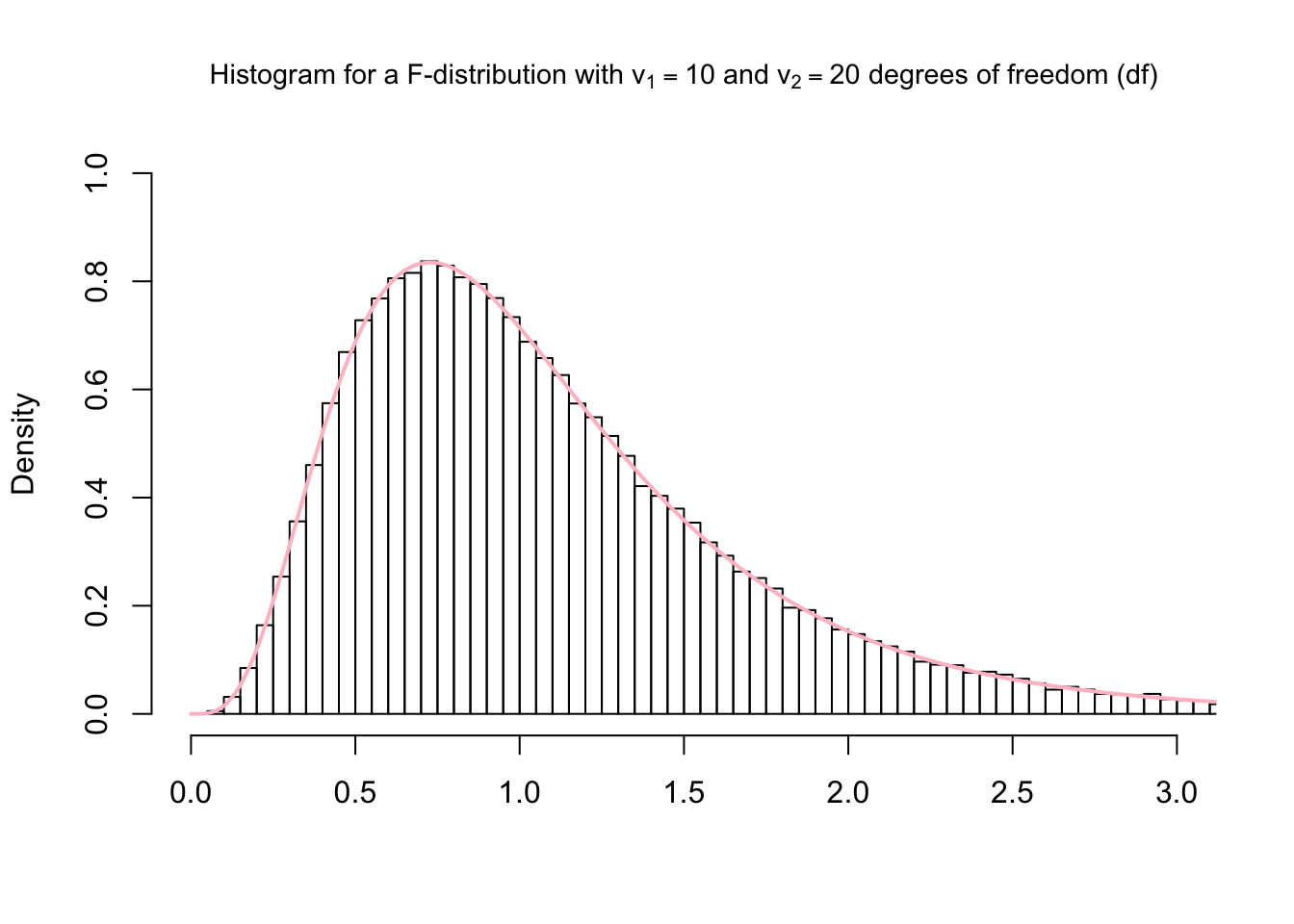The main functions to interact with the $$F$$-distribution are df(), pf(), qf(), rf(). The df() function gives the density, the pf() function gives the distribution function, the qf() function gives the quantile function, and the rf() function generates random deviates.

We use the df() to calculate the density at the value of 1.2 of a $$F$$-curve with $$v_1=10$$ and $$v_2=20$$.

df(1.2, df1 = 10, df2 = 20)
##  0.5626125

We use the pf() to calculate the area under the curve for the interval $$[0,1.5]$$ and the interval $$[1.5, +\infty)$$ of a $$F$$-curve with with $$v_1=10$$ and $$v_2=20$$. Further we ask R if the sum of the intervals $$[0,1.5]$$ and $$[1.5, +\infty)$$ sums up to 1.

x = 1.5
v1 = 10
v2 = 20
# interval $[0,1.5] pf(x, df = v1, df2 = v2, lower.tail = TRUE) ##  0.7890535 # interval$[1.5,+inf)
pf(x, df = v1, df2 = v2, lower.tail = FALSE)
##  0.2109465
pf(x, df = v1, df2 = v2, lower.tail = TRUE) + pf(x, df = v1, df2 = v2, lower.tail = FALSE) == 1
##  TRUE

We use the qf() to calculate the quantile for a given area (= probability) under the curve for a $$F$$-curve with $$v_1=10$$ and $$v_2=20$$ that corresponds to $$q = 0.25, 0.5, 0.75$$ and $$0.999$$. We set lower.tail = TRUE in order the get the area for the interval $$[0, q]$$.

q <- c(0.25, 0.5, 0.75, 0.999)
v1=10
v2=20
qf(q, df1 = v1, df2 = v2, lower.tail = TRUE)
##  0.6563936
qf(q, df1 = v1, df2 = v2, lower.tail = TRUE)
##  0.9662639
qf(q, df1 = v1, df2 = v2, lower.tail = TRUE)
##  1.399487
qf(q, df1 = v1, df2 = v2, lower.tail = TRUE)
##  5.075246

We use rf()function to generate 100,000 random values from the $$F$$-distribution with $$v_1=10$$ and $$v_2=20$$. Thereafter we plot a histogram and compare it to the probability density function of the $$F$$-distribution with $$v_1=10$$ and $$v_2=20$$ (pink line).

x <- rf(100000, df1 = 10, df2 = 20)
hist(x,
breaks = 'Scott',
freq = FALSE,
xlim = c(0,3),
ylim = c(0,1),
xlab = '',
main = (TeX('Histogram for a $\\F$-distribution with $\\v_1 = 10$ and $\\v_2 = 20$ degrees of freedom (df)')), cex.main=0.9)

curve(df(x, df1 = 10, df2 = 20), from = 0, to = 4, n = 5000, col= 'pink', lwd=2, add = T)